## Introduction

One of the most astonishing fundamental observations in cosmology in last decades are the discovery of dark energy and dark matter1,2,3,4,5,6. These two substances of unknown origin comprise about 75% and 20% of the universe’s energy budget5. There may be a dark sector, consisting of particles that do not interact with the known forces (strong, weak, or electromagnetic forces) and the existing observations have demonstrated it. Indeed, many dark sectors could exist, with its own intricate structure, distinct particles and forces. It is often thought that these dark sectors(or “hidden sectors”) may contain new light weakly-coupled particles, particles below the weak-scale that interact with ordinary matter2,7.

At early cosmological times, very light fields in the initial field configuration could lead to dark-matter via coherent oscillations around the minimum of their potential and/or form non-trivial stable field configurations in physical three-dimensional space if their potential allows such a possibility. These non-trivial stable fields, generally referred as topological defects(TDs), are entwined with spontaneous symmetry breakdown8.

As the interaction of TDMs(topological defect dark-matter) with SM can result in some instantaneous changes in some physical properties(eg. mass) of SM elementary particles(e.g. electrons and protons)9,10, their encounter with the Solar System can lead to observable signatures of dark-matter expressed generically in terms of temporary shifts in the frequencies and phases of optical clocks. Observable effects of TDMs can vary greatly, depending on their mass ma. Some literatures on the subject are supplied5, covering a wide range of ma from 10−33 to 105 eV.

In the past two decades, a lot of schemes have been proposed to search DMs, such as the particle physics methods1,3, the laser and maser interferometry method4 and the global network of synchronized atomic magneto-meters method5. A novel detecting mechanism based on frequency-delay system is proposed in this letter. The frequency signal which is generated by an optical clock is sent into a frequency-delay system. After a delay time τ, the signal is compared with the current generated signal. Optical clocks are the most precise instruments in the world11,12,13 and their frequencies are influenced by the change of fine-structure constant(α), they are ideal tools to monitor the change of α thereby detecting the arrival of DMs6,11,14,15. As to the frequency-delay system, long ultra-stable fibre link can be utilized as it is a highly developed precision frequency-transmitting equipment nowadays16. Compared with the reported scheme based on a network of atomic clocks synchronized by high-quality optical fibre links or GPS6, our new method possesses two following advantages:

(1) Long-term synchronization over long distance fibre link of different atomic clocks is suffering too many realistic problems. It is complicated to compare the frequency of two independent atomic clocks accurately for a few years. Instead, two frequency signal with short time difference(ms) of the same atomic clock are directly compared in our scheme, which is more feasible and reliable.

(2) Occasional abrupt changes of fibre link and atomic clock systems may result in misjudgment of dark-matter, which can be avoided by the network of detecting stations. However, the synchronization of atomic clocks system could not eliminate such misjudgments because it is unable to determine which clock is effected by TDMs at the exact time.

## Results

A schematic of TDs detection is shown in Fig. 1. The continuous wavelength (CW) laser is locked to an optical clock via a optical frequency comb. The light of this laser S1 = cos(ωt + ϕ) is sent into a frequency-delay system which is made up by a 1000 km ultra-stable fibre. The fibre is stabilized by a fibre stabilization scheme that will be described in detail in Method Section. In this method, the slow frequency phase noise below the reverse of the round trip delay time 2 × 106/2 × 108 = 10 ms is compensated while the higher frequency phase noise can be controlled by isolating the fibre from the influences such as vibration and so on. The optical power of the transmitted light is boosted by an Er-doped fibre laser (EDFA) every 100 km. After 1000 km the light is retro-reflected with orthogonal polarization by a Faraday mirror. When the retro-reflected light eventually reaches its origin, it has experienced a delay time of τ and becomes S2 = cos[ω(t − τ) + ϕ]. Then it is mixed with S1 and the heterodyne signal Sdetect = cos(ωτ) is obtained. When TDs have not come yet, Sdetect remains constant. However when TDs come, an extra frequency shift ωTD is added to the reference signal S1 due to TDs’ effect on the hyperfine constant α. S1 becomes cos[(ω + ωTD)t]. While the signal S2 has not been effected by the TDs yet, the detecting signal Sdetect is changed into cosTDt + ωτ).The change can be detected and recorded by the phase detector. This change indicates the coming of TDs.

Even though the frequency-delay system has been deliberately designed, a particular problem for a search carried out with a single detector is the appearance of brief spikes in the signal caused by the occasional abrupt(e.g, laser-light-mode jumps and sudden fibre vibration). Rejection of these false-positive signals is difficult for a single detector. Therefore, a network composed of more than five detecting stations, illustrated in Fig. 2, is necessary to reduce the probability of misjudgments of TDMs. Four stations will be used for detecting a TDM and determining its geometrical properties. The other stations will serve as cross-check to verify it, based on predicated TDMs’ arrival, a transient frequency shift of the optical clock in a narrow temporal window.

To make our description more specific, an instance of a domain-wall (2D) network17 is considered. As shown in Fig. 2, the detecting network is composed of n ≥ 5 identical detecting stations (ordinal numbers for 1 to n) separated by distance of Lij(i, j ≤ n) ≥ 300 km. 3D coordinates of four stations and their encountering moments t1 ~ t4 will enable us to unambiguously determine the three-vectors velocity of TDMs. Thus, the narrow window of TDM’s crossing time at the the fifth station, Δt ~ ms, can be predicted to exclude accidental misjudgments.

Supposing τerror and T are corresponding to the average interval time of a single detector misjudgments and the average time between “close encounters” with TDMs. The error decision probability of the detecting network is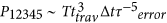, where ttrav ~ Lij/υ ~ s is the travel time of TDMs from station to station. P12345 is less than 10−5 for τerror ~ 100 s and T ≤ 10yrs.

Correlation between signals from multiple, geographically separated detectors can be analyzed by the “excess power” statistic method to distinguish them from noise18. Moreover, increasing the number of stations in the network and the number of detectors of the stations will decrease the error decision probability and admit shorter interval time of single detector misjudgments5. In practice, the network should be composed of the institutions who own optical clocks, such as NIST from U. S., MPI from Germany and SYRTE from France.

## Discussion

In the discussion, a collection of light field beyond SM which can form different types of TDs (monopoles, strings and domain walls) will be introduced. All the light fields are identically considered as ϕ, including both scalar field and vector field. The characteristic transverse size of a defect is determined by the field Compton wavelength d, d ~ ħ/(mac). The mass ma is the typical mass scale of the light field as mentioned above. Only the gross features of TDMs are considered and the amplitude of the field change between inside and outside a TDM is represented as A, A = ϕinside − ϕoutside = ϕ (assuming the outside field to be zero).

From the macroscopic view at distance scales much larger than d, the TDMs can be characterized by its energy density inside the defect, ρinside ~ A2/d2. The network of TDMs will have an additional distance-scale parameter L, the average distance between the defects, which is impossible to calculate without making further assumptions about the mechanisms of TDs formation and evolution.

The energy density of TDM averaged over a large amount of defects is controlled by ρinside and L6: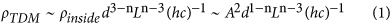and the average time between “close encounters” with TDs is determined by the galactic velocity of such objects υ: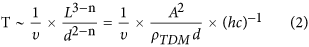The velocity of galactic objects(υg) around the Solar System is well known and for the purpose of estimation, it can taken as υ ~ υg ~ 10−3 × c  ≈ 300 kms−1.

The maximum energy density of the TDMs in the neighborhood of the Solar System is constrained by the experimental estimated dark-matter energy density11, ρDM  0.4 GeV/cm3: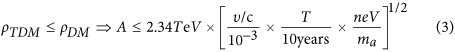This constraint implies some flexible evolution of the TDMs and the possibility for them to build up their mass inside galaxies. The normalization for L and ma in equation (3) is suggested by the requirement of having the average time between “close encounters” is within a few years and of having the signal duration in excess of ~ms.

The duration of the interaction is related to characteristic transverse size and velocity of the defect: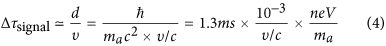Such a crossing time is comparable to the fibre link delay time and in excess of the optical clock self-frequency comparison response time.

We are also interested in how the fields forming the defect interact with the SM, which can be expressed in the form of the quadratic scalar portal,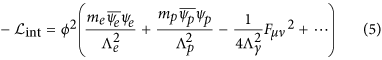where me,p and ψe,p are electron and proton masses and fields and Fμν are electromagnetic tensor components. The appearance of high-energy scales ΛX signifies the effective nature of TDMs, implying that at these scales the scalar portals will be replaced by some unspecified fundamental theory. Equation(5) alter the fundamental constants as follows, respectively,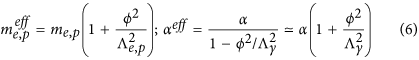Thus, the instantaneous clock frequency shift may be parameterized as: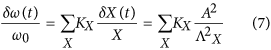where X runs over the fundamental constants. The dimensionless sensitivity coefficients KX are known from atomic and nuclear structure calculations19.

The time difference between the local frequency signal and returning frequency signal from circular fibre link is to be: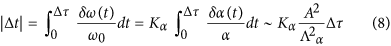where Δτ is the transmission time of circular fibre link, Δτ ~ ms for 200 km fibre link.

The |Δt| and Δτ are combined into a signal factor S = |Δt|/Δτ to be directly compared to experimental sensitivity,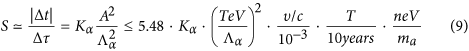where in the inequality the gravitational constraint from equation (3) is used.

The fractional instability of the clock frequency is characterized by Allan variance σy(T). In order to determine the arrival of TDMs, the signal factor S is required to be satisfied S ≥ σy(Δτ), thus,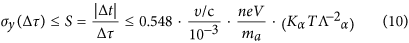and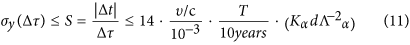where the unit of T, d, Λα are taken to be year(yr), km and TeV. In Fig. 3(a), we plot the experimental accessible parameter space in terms of characteristic time between TDMs, T = L/(10−3c) and the strengthen of coupling constraints, Λα, fixing ma ~ neV, υ/c ~ 10−3. Here we also plot a sensitivity curve to the energy scale Λα as a function of the defect size in Fig. 3(b), fixing the characteristic time between TDMs, T ~ 10yrs. A Sr optical lattice clock (Kα ~ 0.06,σ(ms) ~ 2.1 × 10−17)12 and a Al+ single-ion clock (Kα ~ 0.0078, σ(ms) ~ 2.3 × 10−17)11 are considered in Fig. 3(a,b). The light shaded areas under line (a) and line (b) signify the coupling range that can be realistically probed with these two optical clocks and ultra-stabilized fibre link systems. The dark shaded areas in Fig. 3(b) is the region excluded by the requirement of having the signal duration in excess of ~ms.